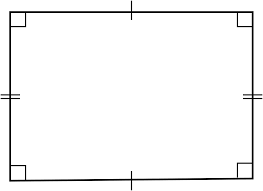# Rectangle Definition: Lesson for Kids

Instructor: Rodney Davis

Rodney has 9 years of elementary teaching experience and a Master of Arts degree in Early Childhood Education.

In this lesson, we'll review the geometric shape of a rectangle. We'll discuss the definition and characteristics of rectangles. You'll also learn how to tell the difference between rectangles and other four-sided shapes.

## What is a Rectangle?

Saturday is finally here! Your dad promised you that on Saturday he would take you and your little sister to the college football game. After you take your seats, your sister asks you ''what the shape of the field is?'' You begin to take a closer look at the football field, noticing for the first time that the field is in the shape of a rectangle. So you try to explain to her, what a rectangle is? A rectangle is a four-sided polygon containing four right angles.## Angles of Rectangles

In order to understand a right angle, we must know what an angle is. An angle is a geometric term for when two rays, or lines, meet and form a point, like the letter v. A perfect example of a right angle would be a capital L. In right angles, the measurement of the angle is always 90 degrees. So, rectangles have four right angles and four sides. The opposite sides of a rectangle are the same length, which is called congruent.

## Measuring Rectangles

We already learned that rectangles must have four sides with four right angles. But, not every rectangle is the same size, even though, they have the same characteristics. While both a football field and a sheet of paper are rectangles, we know they are very different sizes! So, how do we measure rectangles?

#### Area

Every rectangle has an area and perimeter. Area is the measurement inside of the rectangle. It's the size of the whole surface of the rectangle. Area is calculated by multiplying the length (how long the rectangle is) by the width (how wide the rectangle is). The area is always measured in square units.

• Area = length * width

If a rectangle has a length of 5 inches and width of 8 inches. What is the area?

• Area = 5 * 8

The area would equal 40 square inches.

#### Perimeter

The perimeter measures the distance around the rectangle. Again if a rectangle has a length of 5 inches (how long the rectangle is) and a width of 8 inches (how wide the rectangle is). To find the perimeter, we want to add all four sides together.

• Perimeter = length + length + width + width

To unlock this lesson you must be a Study.com Member.

### Register to view this lesson

Are you a student or a teacher?

#### See for yourself why 30 million people use Study.com

##### Become a Study.com member and start learning now.
Back
What teachers are saying about Study.com

### Earning College Credit

Did you know… We have over 200 college courses that prepare you to earn credit by exam that is accepted by over 1,500 colleges and universities. You can test out of the first two years of college and save thousands off your degree. Anyone can earn credit-by-exam regardless of age or education level.